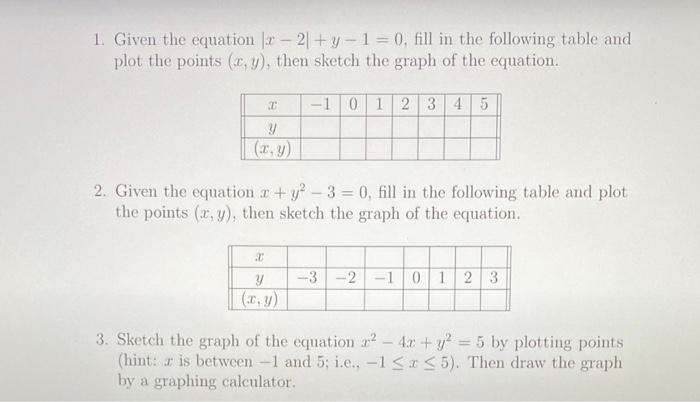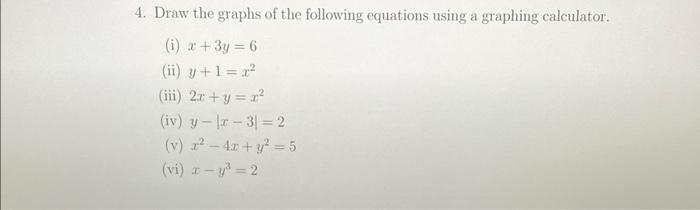Home / Expert Answers / Algebra / need-help-1-given-the-equation-x-2-y-1-0-fill-in-the-following-table-and-plot-the-points-pa284

# (Solved): need help! 1. Given the equation $$|x-2|+y-1=0$$, fill in the following table and plot the points ...

need help!1. Given the equation $$|x-2|+y-1=0$$, fill in the following table and plot the points $$(x, y)$$, then sketch the graph of the equation. 2. Given the equation $$x+y^{2}-3=0$$, fill in the following table and plot the points $$(x, y)$$, then sketch the graph of the equation. 3. Sketch the graph of the equation $$x^{2}-4 x+y^{2}=5$$ by plotting points (hint: $$x$$ is between $$-1$$ and 5 ; i.e., $$-1 \leq x \leq 5$$ ). Then draw the graph by a graphing calculator. 4. Draw the graphs of the following equations using a graphing calculator. (i) $$x+3 y=6$$ (ii) $$y+1=x^{2}$$ (iii) $$2 x+y=x^{2}$$ (iv) $$y-|x-3|=2$$ (v) $$x^{2}-4 x+y^{2}=5$$ (vi) $$x-y^{3}=2$$

We have an Answer from Expert